Question

# Draw the Lewis structure of XeCl. Include all lone pairs. Identify the molecular geometry of Xeci,...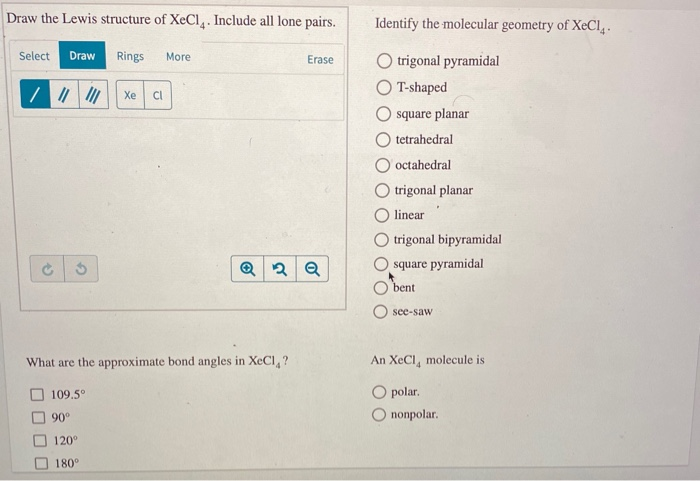Draw the Lewis structure of XeCl. Include all lone pairs. Identify the molecular geometry of Xeci, Select Draw Rings More Erase trigonal pyramidal T-shaped square planar O tetrahedral O octahedral trigonal planar linear trigonal bipyramidal square pyramidal bent O see-saw What are the approximate bond angles in XeCl,? An XeCl, molecule is O polar O nonpolar. 109.5° 90° 120° 180°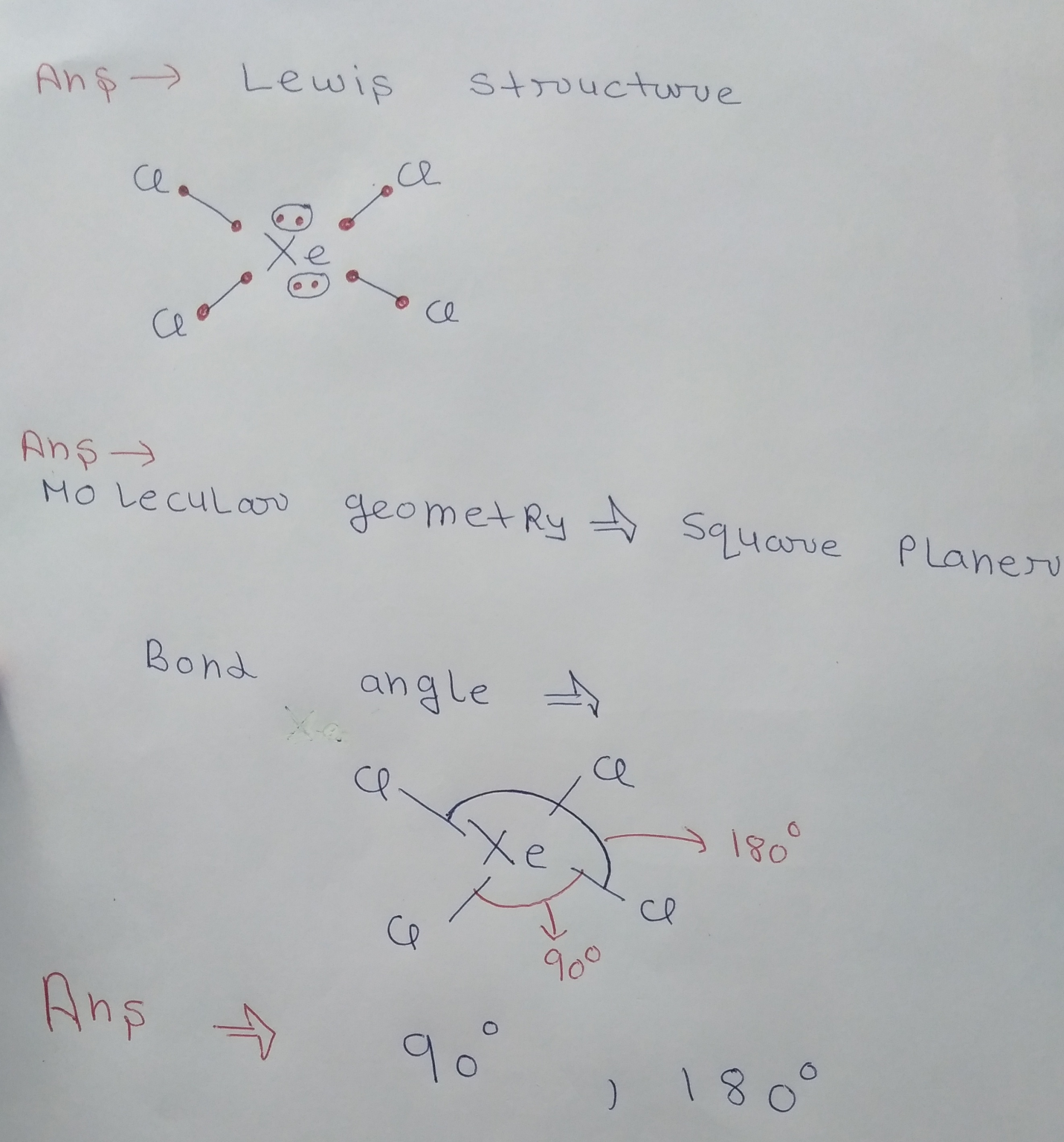#### Earn Coins

Coins can be redeemed for fabulous gifts.

Similar Homework Help Questions
• ### Draw the Lewis structure of CIBr, showing all lone pairs. Identify the molecular geometry of CIBry...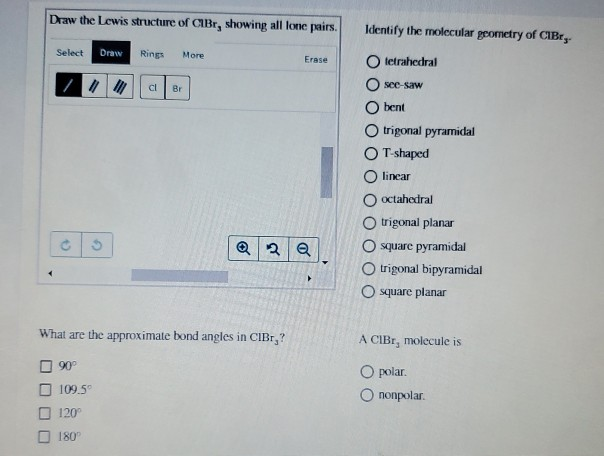Draw the Lewis structure of CIBr, showing all lone pairs. Identify the molecular geometry of CIBry Select Draw Rings More Erase tetrahedral see-saw bent trigonal pyramidal T-shaped O linear octahedral trigonal planar O square pyramidal trigonal bipyramidal square planar What are the approximate bond angles in CIBr,? A CIBr, molecule is O polar. Ononpolar. 90° 109.5 120 180

• ### Draw the Lewis structure of SF 4 showing all lone pairs.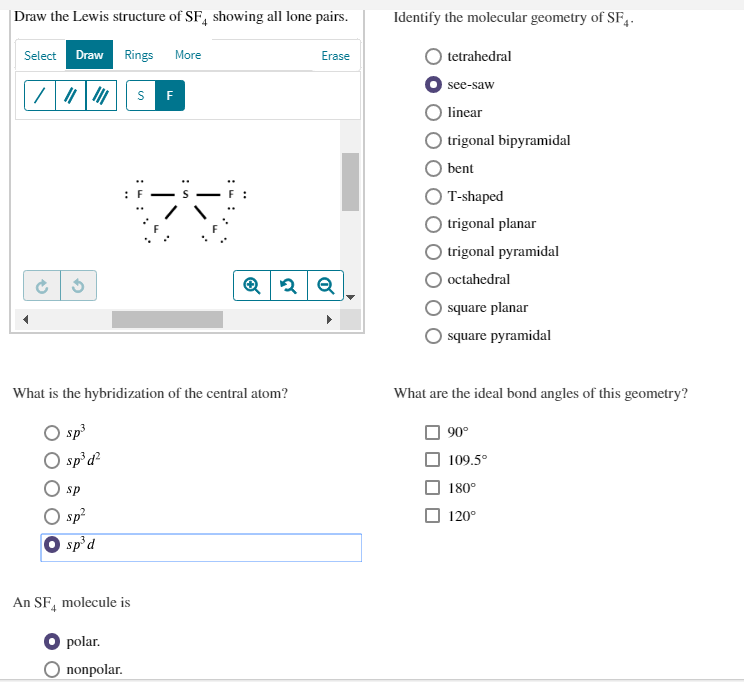Draw the Lewis structure of $$\mathrm{SF}_{4}$$ showing all lone pairs.Identify the molecular geometry of $$\mathrm{SF}_{4}$$.tetrahedralsee-sawlineartrigonal bipyramidalbentT-shapedtrigonal planartrigonal pyramidaloctahedralsquare planarsquare pyramidal$$\begin{array}{l} \text { What is the hybridization of the central atom? }\\ s p^{3}\\ s p^{3} d^{2}\\ s p\\ s p^{2}\\ \text s p^{3} d \end{array}$$$$\begin{array}{l} \text { What are the ideal bond angles of this geometry? }\\ 90^{\circ}\\ 109.5^{\circ}\\ 180^{\circ}\\ 120^{\circ} \end{array}$$An $$\mathrm{SF}_{4}$$ molecule ispolar.nonpolar.

• ### Draw the Lewis structure of AsO 4 3− ​ showing all lone pairs.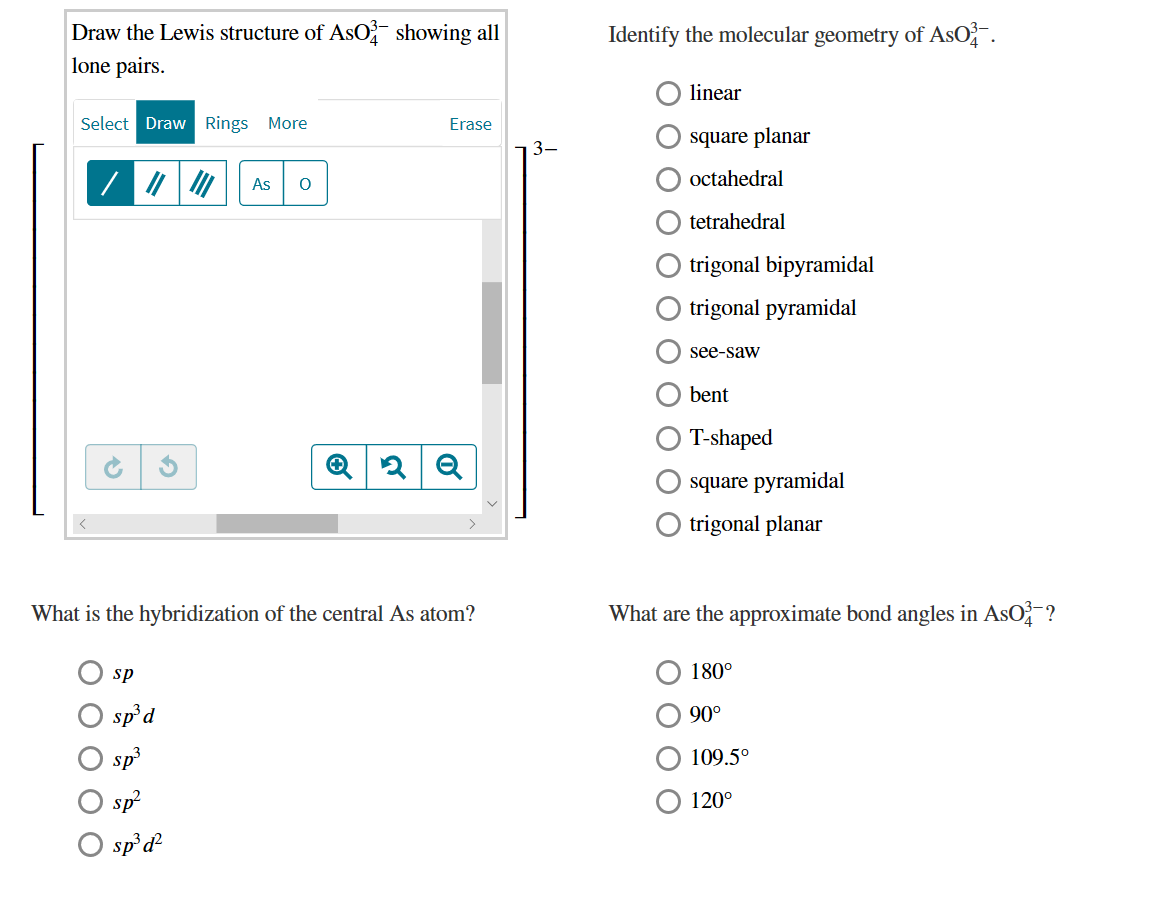Draw the Lewis structure of $$\mathrm{AsO}_{4}^{3-}$$ showing all lone pairs.Identify the molecular geometry of $$\mathrm{AsO}_{4}^{3-}$$.linearsquare planaroctahedraltetrahedraltrigonal bipyramidaltrigonal pyramidalsee-sawbentT-shapedsquare pyramidaltrigonal planar$$\begin{array}{l} \text { What is the hybridization of the central As atom? }\\ s p\\ s p^{3} d\\ s p^{3}\\ s p^{2}\\ s p^{3} d^{2} \end{array}$$$$\begin{array}{l} \text { What are the approximate bond angles in } \mathrm{AsO}_{4}^{3-} \text { ? }\\ 180^{\circ}\\ 90^{\circ}\\ 109.5^{\circ}\\ 120^{\circ} \end{array}$$

• ### Provide the correct molecular geometry for (c), given the number of lone pairs and bonding groups...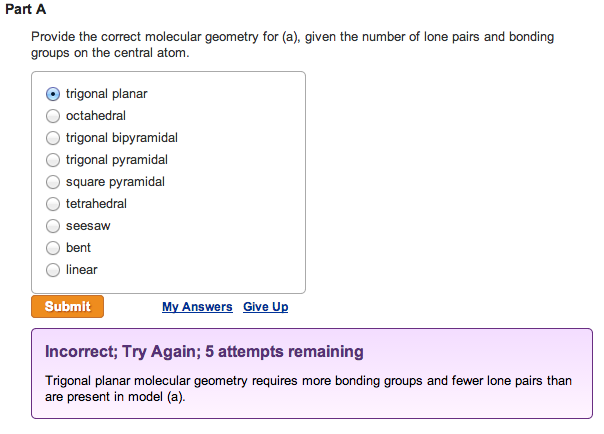Provide the correct molecular geometry for (a), given the number of lone pairs and bonding groups on the central atom. trigonal planar  octahedral  trigonal bipyramidal  trigonal pyramidal  square pyramidal  tetrahedral  seesaw  bent  linearProvide the correct molecular geometry for (b), given the number of lone pairs and bonding groups on the central atom.  trigonal bipyramidal  square pyramidal  bent  tetrahedral  trigonal planar  seesaw  trigonal pyramidal  octahedral  linear Provide the correct molecular geometry for (c), given the number of lone pairs and bonding groups on the...

• ### Draw the Lewis structure for AsH₃, then answer the following questions:

Draw the Lewis structure for AsH₃, then answer the following questions: A. How many valence electrons does this compound have? B. How many bonded electrons does this compound have? C. How many lone pairs of electrons does this compound have? D. How many single bonds does this compound have? E. How many double bonds does this compound have? F. What is the electron group arrangement {aka electron pair geometry}(enter one of the following: linear, trigonal planar, tetrahedral, trigonal bipyramidal, octahedral)? G. What is the molecular geometry {aka...

• ### Number of Electron Groups Ideal Bond Angles VSEPR Molecular Shape Answer Bank bent Bonded Atoms/ Lone...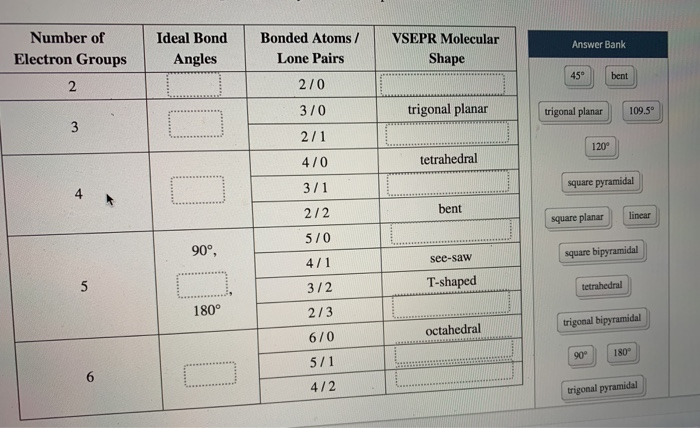Number of Electron Groups Ideal Bond Angles VSEPR Molecular Shape Answer Bank bent Bonded Atoms/ Lone Pairs 270 370 2/1 trigonal planar trigonal planar 109.5 120 470 tetrahedral 3/1 square pyramidal bent square planar linear 90° square bipyramidal 2/2 570 4/1 3/2 2/3 see-saw T-shaped tetrahedral trigonal bipyramidal octahedral 6/0 5/1 4/2 trigonal pyramidal Which of the substances has polar interactions (dipole-dipole forces) between molecules? HF Cci, CHCI, so, tu a m carrers privacy polic

• ### Specify the electron-domain geometry for the following two species using the VSEPR model: KrCl4 and BrO4....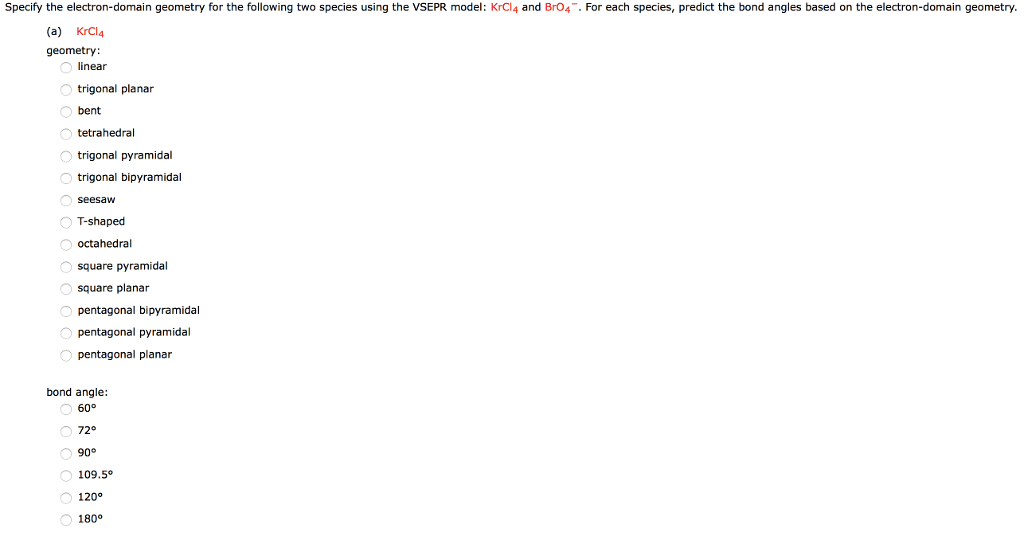Specify the electron-domain geometry for the following two species using the VSEPR model: KrCl4 and BrO4. For each species, predict the bond angles based on the electron-domain geometry. (a) KrCl4 geometry linear trigonal planar bent tetrahedral trigonal pyramidal trigonal bipyramidal T-shaped octahedral square pyramidal square planar pentagonal bipyramidal pentagonal pyramidal pentagonal planar bond angle: 72° 109.5° 120 180° (b) Br04 geometry: inear trigonal planar bent tetrahedral trigonal pyramidal trigonal bipyramidal seesaW T-shaped octahedral square pyramidal square planar pentagonal bipyramidal pentagonal...

• ### 10.) Specify the electron-domain geometry for the following two species using the VSEPR model: Xe...

10.) Specify the electron-domain geometry for the following two species using the VSEPR model: XeCl4 and IO4−. For each species, predict the bond angles based on the electron-domain geometry. (a)    XeCl4 geometry: linear trigonal planar    bent tetrahedral trigonal pyramidal trigonal bipyramidal seesaw T-shaped octahedral square pyramidal square planar pentagonal bipyramidal pentagonal pyramidal pentagonal planar bond angle: 60° 72°   90° 109.5° 120° 180° (b)    IO4− geometry: linear trigonal planar    bent tetrahedral trigonal pyramidal trigonal bipyramidal seesaw T-shaped octahedral square pyramidal square...

• ### Draw the Lewis structure for PCl₆-, then answer the following questions:

Draw the Lewis structure for PCl₆-, then answer the following questions: A. How many valence electrons does this compound have? B. How many bonded electrons does this compound have? C. How many lone pairs of electrons does this compound have? D. How many single bonds does this compound have? E. How many double bonds does this compound have? F. What is the electron group arrangement {aka electron pair geometry} (enter one of the following: linear, trigonal planar, tetrahedral, trigonal bipyramidal, octahedral)? G. What is the molecular geometry {aka...

• ### VSEPR theory predicts which shape for the molecular structure of the following molecules or molecular ions?...

VSEPR theory predicts which shape for the molecular structure of the following molecules or molecular ions? (i.e. the arrangement of only the bonded atoms about the central atom) bent (or angular) linear octahedral see-saw square planar square pyramidal tetrahedral trigonal bipyramidal trigonal planar trigonal pyramidal T-shaped  ICl3 molecule    (The central atom is I.) bent (or angular) linear octahedral see-saw square planar square pyramidal tetrahedral trigonal bipyramidal trigonal planar trigonal pyramidal T-shaped  IO4- ion    (The central atom is I.) bent (or angular) linear...